Math 321 (981)

Introduction to Numerical Computing

Assignment # 1

Due: Wed September 23, 1:00 p.m.

You can indicate subscripts and superscripts using the notations a_i, a^i or Matlab syntax.

1. P1.1, #2.
2. P1.1, #7 (a, c). Write a Matlab script for each.
3. CP1.1, #6.
4. A. Write a Matlab script for evaluating a polynomial of any degree using nested multiplication. The input is to be obtained from the user during M-file execution.
5. B. Count the number of arithmetic operations. Compare with the count from the Matlab function flops.

6. Count the number of operations needed in multiplying two square matrices. Compare with the count from the function flops.
7. Write a Matlab script for calculating the derivative of the function cosh x. Compare the result of your code with the value from Matlab built-in functions.

Assignment # 2

Due: Mon October 5, 1:00 p.m.

Problem (1)

Consider the function and its derivative:

y = (x+3.11) * (x -.99)^2;

y = x^3 + 113/100*x^2 - 51777/10000*x + 3048111/1000000;

yp = 3*x^2 + 113/50*x - 51777/10000;

Use the function ezplot to sketch the function. The syntax could be

ezplot(' expression ') or fplot('f',[a b])

(a) From the graph, give a value that is very close to each root.

(b) For each root give the following information using tol = 1e-10.

 Method Starting values No. of iterations Calculated value Bisection Newton Secant

Problem (2)

What is the stopping criterion used in fzero function.

Problem (3)

Consider the function

y = (x^3 + 2.3*x^2 - .85*x - 3.179)/ x^3

with derivative

yp = (-2.300*x^2 + 1.700*x + 9.537) / x^4;

This function has the two exact roots:

r1 = 1.1, r2 = -1.7

For r1 answer the following. Give the calling statement of the functions for each.

(a) Give data that supports the quadratic convergence of Newton’s method.

(b) Give data that supports that Bisection method does not have linear convergence.

(c) If you start Newton’s method with x0 = 1.5, the first few steps do not demonstrate quadratic convergence, does this contradict the results about the method? Explain.

For r2 answer the following:

(d) Does Newton’s method converges quadratically to r2? Explain.

(e) Describe the convergence when the formula x_n+1 = x_n — m f_n / f’_n is used for m = 2, 3 and 4.

Assignment # 3

Due: Mon October 19.

Problem (1)

Consider the data

 X 7.92 9.22 7.4 1.77 4.05 9.35 9.17 4.1 8.93 1 3.52 Y -4.9829 -0.7054 0.2119 -1.4692 -0.4422 -0.2282 -0.9587 -0.2729 -11.5369 -0.1425 2.1488

a) Use the matlab function "divdif" to construct the divided-difference table.

b) What are the coefficients, ai, for Newton interpolating polynomial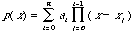?

(Hint. Use the function "diag")

c) What is the power and coefficient of the leading term of the polynomial?

Problem (2).

Use "divdif" to show that a polynomial of degree 4 can represent the data.

 X 0.2 1.6 0.5 1 -2 1.1 1.5 Y 0.4016 2.7536 -0.4375 -1 23 -0.8359 1.5625

Problem (3)

Consider the function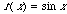.

(a) Use the function "richard" to calculate the quantities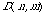,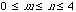, for.

(b) Discuss the accuracy of the calculated quantities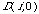.

(c) Compare the accuracy of the calculated quantitieswith the results of the book.

Problem (4)

(a) Write and email a function "richard2" similar to "richard" that computes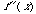with Richardson extrapolation using the formula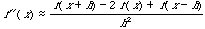.

(b) Use the function "richard2" to calculate the quantities,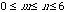, that approximate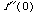for. Present the results.

Problem (5). Ex 4 page 136.

Problem (6). Ex 7 page 149.

Problem (7). Ex 11 page 150.

Problem (8). Ex 3 page 161.